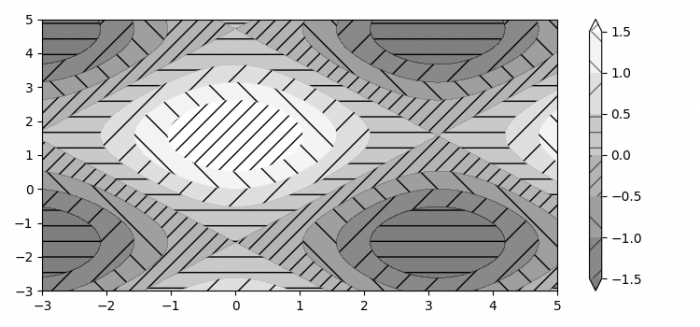# Contour hatching in Matplotlib plot

MatplotlibPythonData Visualization

#### Python Data Science basics with Numpy, Pandas and Matplotlib

Most Popular

63 Lectures 6 hours

#### Data Visualization using MatPlotLib & Seaborn

11 Lectures 4 hours

#### MatPlotLib with Python

9 Lectures 2.5 hours

To plot contour with hatching, we can take the following steps −

• Set the figure size and adjust the padding between and around the subplots.
• Create x, y and z data points using numpy.
• Flat the x and y data points.
• Create a figure and a set of subplots.
• Plot a contour with different hatches.
• Create a colorbar for a scalar mappable instance.
• To display the figure, use show() method.

## Example

import matplotlib.pyplot as plt
import numpy as np

plt.rcParams["figure.figsize"] = [7.50, 3.50]
plt.rcParams["figure.autolayout"] = True

x = np.linspace(-3, 5, 150).reshape(1, -1)
y = np.linspace(-3, 5, 120).reshape(-1, 1)
z = np.cos(x) + np.sin(y)

x, y = x.flatten(), y.flatten()

fig1, ax1 = plt.subplots()

cs = ax1.contourf(x, y, z, hatches=['-', '/', '\', '//'],
cmap='gray', extend='both', alpha=0.5)
fig1.colorbar(cs)

plt.show()

## Output